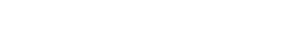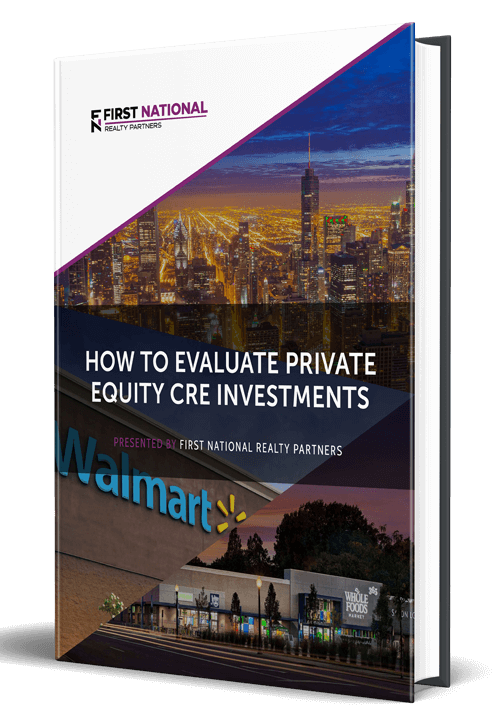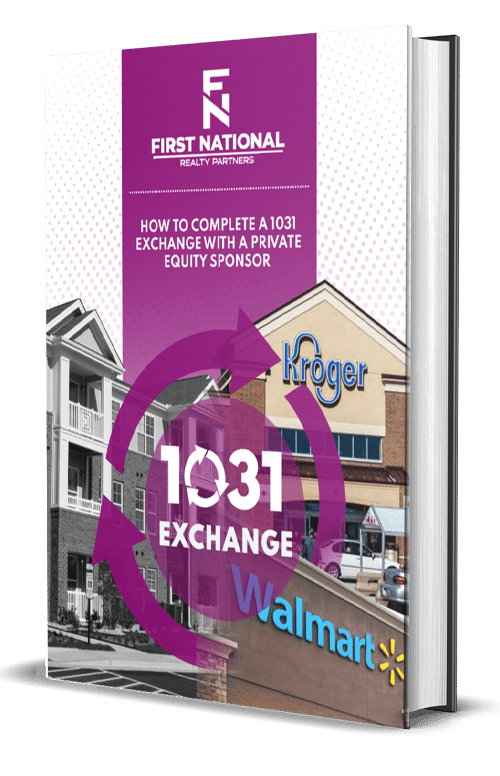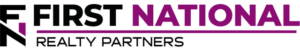# How To Calculate Net Operating Income Using The Cap Rate

### Key Takeaways

• Net Operating Income is a real estate investment metric that measures a property’s operational efficiency/profitability.  It is calculated as total gross rents minus operating expenses.
• The cap rate is both a measure of risk and return.  It is calculated by dividing net operating income by the property’s value.  But, these variables aren’t always known when evaluating a potential investment.
• Of the three variables in the cap rate equation, cap rate, NOI, and value, one of them can be calculated as long as the other two are known.  For example, if a real estate investor is trying to solve for NOI, they can do so by multiplying the cap rate by the estimated value.
• Having an understanding of each of these variables, can also help real estate investors understand the potential return on a commercial real estate investment.

Real estate investors use a variety of metrics to measure the performance of a commercial real estate investment.  Although they each require their own set of inputs to calculate, many of them are related.  This is the case with Net Operating Income (NOI) and the Capitalization Rate (Cap Rate).

In this article, we will define Net Operating Income and Cap Rate.  In addition, we will illustrate their relationship to one another by demonstrating how to calculate one by using the other as an input.  By the end, readers will have a better understanding of the relationship between these two metrics and why they are critically important to measuring the performance of an investment property.

At First National Realty Partners, we always calculate the Cap Rate and Net Operating Income as part of our pre-investment due diligence.  We believe that these are critical indicators of an investment’s potential rate of return and we only present properties to our investors when they have passed a rigorous testing process, which includes these two metrics.  To learn more about our current investment opportunities, click here

## Net Operating Income and Cap Rate Defined

In order to explain the relationship between these two metrics, it helps to start with a definition of each.

### What is Net Operating Income?

Net Operating Income (NOI) is a performance metric that measures the operational efficiency of a property.  It is calculated as:

Net Operating Income:  Gross Income – Operating Expenses

In this equation, Gross Income represents a property’s total income from all sources including rental income, fee income, interest income, and “other” income.  Operating expenses represent the costs that are needed to run a property on a day to day basis.  For most properties, these include:  property taxes, property management, insurance, maintenance, utilities, legal, and admin.

For example, if a property has \$500,000 in gross income and \$250,000 in operating expenses, the resulting NOI would be \$250,000.

Commercial properties are valued based on the amount of net operating income they produce, but NOI is only one input in the valuation equation.  The other is the cap rate.

### What is the Cap Rate?

The Cap Rate is a real estate metric that explains the relationship between a rental property’s net operating income and market value/purchase price.  In commercial real estate investing, it has two functions.  It is a measure of a property’s potential return on investment, assuming it was purchased with cash.  And, it can be used as a way to indicate the risk associated with an asset.  A lower cap rate signals less risk and a higher cap rate signals higher risk.

The cap rate formula is:

Cap Rate = Net Operating Income / Property Value

Breaking down these inputs, NOI is calculated as gross income minus operating expenses (as described above).  The value of a property is most commonly represented by its purchase price or its market value as determined by a third party appraiser.   But, the challenge with the cap rate calculation is that both of these  variables aren’t always known when evaluating a potential investment.  For example, a real estate investor may know NOI, but not the value.  Fortunately, the cap rate formula can be rearranged to solve for a missing variable when necessary.

## How To Calculate NOI Using the Cap Rate

There are three variables in the cap rate formula above, Cap Rate, NOI, and Property Value.  If two of the three are known, the third can be solved for.  For example, as it relates to the title of this article, NOI can be calculated when a property’s cap rate and value are known.  The rearranged equation looks like this:

NOI = Cap Rate * Property Value

In this example, the cap rate can be obtained from reviewing recent sales of comparable properties in the same real estate market and the value of the property can be obtained from the purchase price, asking price, appraised value, or an estimate of current market value.

To illustrate this point, suppose a property has a known value of \$1,000,000 and a cap rate of 7%.  With this information, the annual NOI could be estimated at \$70,000.

## Summary & Conclusions

Net Operating Income is a real estate investment metric that measures a property’s operational efficiency/profitability.  It is calculated as total gross rents minus operating expenses.

The cap rate is both a measure of risk and return.  It is calculated by dividing net operating income by the property’s value.  But, these variables aren’t always known when evaluating a potential investment.

Of the three variables in the cap rate equation, cap rate, NOI, and value, one of them can be calculated as long as the other two are known.  For example, if an investor is trying to solve for NOI, they can do so by multiplying the cap rate by the estimated value.

Having an understanding of each of these variables can also help investors understand the potential return on a commercial real estate investment.

## Interested In Learning More?

First National Realty Partners is one of the country’s leading private equity commercial real estate investment firms. With an intentional focus on finding world-class, multi-tenanted assets well below intrinsic value, we seek to create superior long-term, risk-adjusted returns for our investors while creating strong economic assets for the communities we invest in.

Get instant access to all of our current and past commercial real estate deals.## Subscribe Now

Get the latest news on real estate

1031 Exchange

Acquisitions

CRE Basics

## Get More From FNRP## How to Evaluate Private Equity CRE Investments## How to Complete a 1031 Exchange with a Private Equity Sponsor